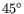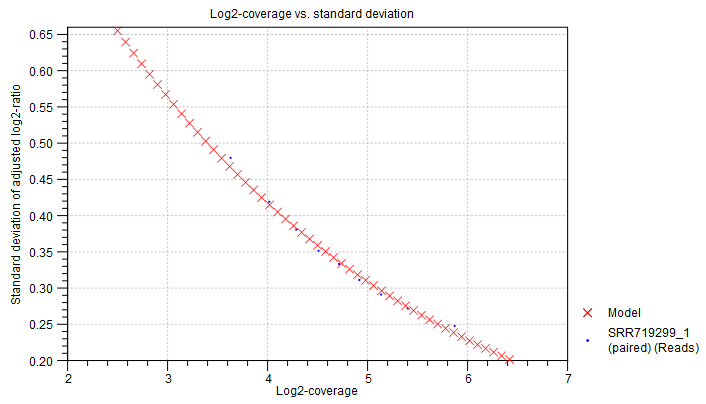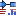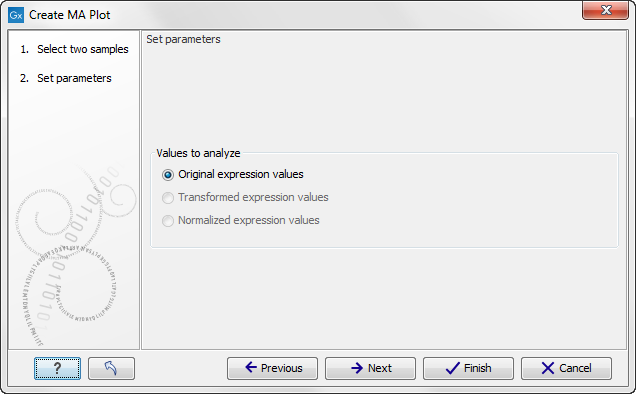## MA plot

The MA plot is a scatter rotated by. For two samples of expression values it plots for each gene the difference in expression against the mean expression level. MA plots are often used for quality control, in particular, to assess whether normalization and/or transformation is required.

You can create an MA plot comparing two samples:

Toolbox | Transcriptomics Analysis ()| General Plots | Create MA Plot ()

Select two samples ( (), () or ()). Clicking Next will display a dialog as shown in figure 26.104.Figure 26.104: Selcting which values the MA plot should be based on.

In this dialog, you select the values to be used for creating the MA plot (see Selecting transformed and normalized values for analysis).

Click Next if you wish to adjust how to handle the results. If not, click Finish.

Subsections